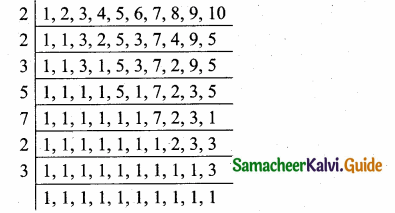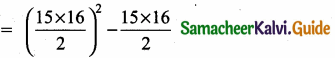Students can download Maths Chapter 2 Numbers and Sequences Ex 2.10 Questions and Answers, Notes, Samacheer Kalvi 10th Maths Guide Pdf helps you to revise the complete Tamilnadu State Board New Syllabus, helps students complete homework assignments and to score high marks in board exams.

## Tamilnadu Samacheer Kalvi 10th Maths Solutions Chapter 2 Numbers and Sequences Ex 2.10

Multiple choice questions:

Question 1.
Euclid’s division lemma states that for positive integers a and b, there exist unique integers q and r such that a = bq + r, where r must satisfy ………………….
(1) 1 < r < b
(2) 0 < r < b
(3) 0 < r < 6
(4) 0 < r < b
Ans.
(3) 0 < r < bQuestion 2.
Using Euclid’s division lemma, if the cube of any positive integer is divided by 9 then the possible remainders are ………………….
(1) 0, 1, 8
(2) 1, 4, 8
(3) 0, 1, 3
(4) 1, 3, 5
(1) 0, 1, 8
Hint: Let the +ve integer be 1, 2, 3, 4 …………
13 = 1 when it is divided by 9 the remainder is 1.
23 = 8 when it is divided by 9 the remainder is 8.
33 = 27 when it is divided by 9 the remainder is 0.
43 = 64 when it is divided by 9 the remainder is 1.
53 = 125 when it is divided by 9 the remainder is 8.
The remainder 0, 1, 8 is repeated.

Question 3.
If the H.C.F of 65 and 117 is expressible in the form of 65m – 117 , then the value of m is
(1) 4
(2) 2
(3) 1
(4) 3
(2) 2
Hint:
H.C.F. of 65 and 117
117 = 65 × 1 + 52
65 = 52 × 1 + 13
52 = 13 × 4 + 0
∴ 13 is the H.C.F. of 65 and 117.
65m – 117 = 65 × 2 – 117
130 – 117 = 13
∴ m = 2Question 4.
The sum of the exponents of the prime factors in the prime factorization of 1729 is …………………….
(1) 1
(2) 2
(3) 3
(4) 4
(3) 3
Hint: 1729 = 7 × 13 × 19
Sum of the exponents = 1 + 1 + 1
= 3

Question 5.
The least number that is divisible by all the numbers from 1 to 10 (both inclusive) is
(1) 2025
(2) 5220
(3) 5025
(4) 2520
(4) 2520
Hint:L.C.M. = 23 × 32 × 5 × 7
= 8 × 9 × 5 × 7
= 2520Question 6.
74k ≡ ______ (mod 100)
(1) 1
(2) 2
(3) 3
(4) 4
(1) 1
Hint:
74k ≡______ (mod 100)
y4k ≡ y4 × 1 = 1 (mod 100)

Question 7.
Given F1 = 1 , F2 = 3 and Fn = Fn-1 + Fn-2 then F5 is ………….
(1) 3
(2) 5
(3) 8
(4) 11
(4) 11
Hint:
Fn = Fn-1 + Fn-2
F3 = F2 + F1 = 3 + 1 = 4
F4 = F3 + F2 = 4 + 3 = 7
F5 = F4 + F3 = 7 + 4 = 11Question 8.
The first term of an arithmetic progression is unity and the common difference is 4. Which of the following will be a term of this A.P
(1) 4551
(2) 10091
(3) 7881
(4) 13531
(3) 7881
Hint:
t1 = 1
d = 4
tn = a + (n – 1)d
= 1 + 4n – 4
4n – 3 = 4551
4n = 4554
n = will be a fraction
It is not possible.
4n – 3 = 10091
4n = 10091 + 3 = 10094
n = a fraction
4n – 3 = 7881
4n = 7881 + 3 = 7884
n = $$\frac{7884}{4}$$, n is a whole number.
4n – 3 = 13531
4n = 13531 – 3 = 13534
n is a fraction.
∴ 7881 will be 1971st term of A.P.Question 9.
If 6 times of 6th term of an A.P is equal to 7 times the 7th term, then the 13th term of the A.P. is ………..
(1) 0
(2) 6
(3) 7
(4) 13
(1) 0
Hint:
6 t6 = 7 t7
6(a + 5d) = 7 (a + 6d) ⇒ 6a + 30d = 7a + 42d
30 d – 42 d = 7a – 6a ⇒ -12d = a
t13 = a + 12d (12d = -a)
= a – a = 0

Question 10.
An A.P consists of 31 terms. If its 16th term is m, then the sum of all the terms of this A.P. is
(1) 16 m
(2) 62 m
(3) 31 m
(4) $$\frac { 31 }{ 2 }$$ m
(3) 31 m
Hint:
t16 = m
S31 = $$\frac { 31 }{ 2 }$$ (2a + 30d)
= $$\frac { 31 }{ 2 }$$ (2(a + 15d))
(∵ t16 = a + 15d)
= 31(t16) = 31mQuestion 11.
In an A.P., the first term is 1 and the common difference is 4. How many terms of the A.P must be taken for their sum to be equal to 120?
(1) 6
(2) 7
(3) 8
(4) 9
(3) 8
Here a = 1, d = 4, Sn = 120
Sn = $$\frac { n }{ 2 }$$[2a + (n – 1)d]
120 = $$\frac { n }{ 2 }$$ [2 + (n – 1)4] = $$\frac { n }{ 2 }$$ [2 + 4n – 4)]
= $$\frac { n }{ 2 }$$ [4n – 2)] = $$\frac { n }{ 2 }$$ × 2 (2n – 1)
120 = 2n2 – n
∴ 2n2 – n – 120 = 0 ⇒ 2n2 – 16n + 15n – 120 = 0
2n(n – 8) + 15 (n – 8) = 0 ⇒ (n – 8) (2n + 15) = 0
n = 8 or n = $$\frac { -15 }{ 2 }$$ (omitted)
∴ n = 8

Question 12.
A = 265 and B = 264 + 263 + 262 …. + 20 which of the following is true?
(1) B is 264 more than A
(2) A and B are equal
(3) B is larger than A by 1
(4) A is larger than B by 1
(4) A is larger than B by
A = 265
B = 264+63 + 262 + …….. + 20
= 2
= 1 + 22 + 22 + ……. + 264
a = 1, r = 2, n = 65 it is in G.P.
S65 = 1 (265 – 1) = 265 – 1
A = 265 is larger than BQuestion 13.
The next term of the sequence $$\frac { 3 }{ 16 }$$,$$\frac { 1 }{ 8 }$$,$$\frac { 1 }{ 12 }$$,$$\frac { 1 }{ 18 }$$ is ………..
(1) $$\frac { 1 }{ 24 }$$
(2) $$\frac { 1 }{ 27 }$$
(3) $$\frac { 2 }{ 3 }$$
(4) $$\frac { 1 }{ 81 }$$
(2) $$\frac { 1 }{ 27 }$$
Hint:
$$\frac { 3 }{ 16 }$$,$$\frac { 1 }{ 8 }$$,$$\frac { 1 }{ 12 }$$,$$\frac { 1 }{ 18 }$$
a = $$\frac { 3 }{ 16 }$$, r = $$\frac { 1 }{ 8 }$$ ÷ $$\frac { 3 }{ 16 }$$ = $$\frac { 1 }{ 8 }$$ × $$\frac { 16 }{ 3 }$$ = $$\frac { 2 }{ 3 }$$
The next term is = $$\frac { 1 }{ 18 }$$ × $$\frac { 2 }{ 3 }$$ = $$\frac { 1 }{ 27 }$$

Question 14.
If the sequence t1,t2,t3 … are in A.P. then the sequence t6,t12,t18 … is
(1) a Geometric Progression
(2) an Arithmetic Progression
(3) neither an Arithmetic Progression nor a Geometric Progression
(4) a constant sequence
(2) an Arithmetic Progression
Hint:
If t1, t2, t3, … is 1, 2, 3, …
If t6 = 6, t12 = 12, t18 = 18 then 6, 12, 18 … is an arithmetic progressionQuestion 15.
The value of (13 + 23 + 33 + ……. + 153) – (1 + 2 + 3 + …….. + 15) is …………….
(1) 14400
(2) 14200
(3) 14280
(4) 14520